# Common Data Transformations

It's often useful in performing data analysis to transform some of your variables to fit a common scale; this is especially useful in exploratory data analysis, because these transformations often make it much easier to eyeball the relationship between variables. (Also, some statistical techniques require these transformations.)

In this short lesson, we'll introduce two common methods of transforming data---the log transform and centering and scaling (a.k.a. standardizing), and we'll look at examples of them through the data underlying part of my 2016 book, The Rule of Law in the Real World (shameless plug).

Let's start by loading the data and taking a quick look at what it looks like, as well as setting up the libraries we'll need.

In :
import pandas as pd
import seaborn as sns
%matplotlib inline

/Users/pg/.local/share/virtualenvs/library_gobbledygook-NjdFyGcL/lib/python3.6/site-packages/matplotlib/__init__.py:886: MatplotlibDeprecationWarning:
examples.directory is deprecated; in the future, examples will be found relative to the 'datapath' directory.
"found relative to the 'datapath' directory.".format(key))

In :
df = pd.read_csv("http://rulelaw.net/downloads/rol-scores.csv")

In :
df.head()

Out:
State Pop. In Millions for 2012 RoLScore elec_pros pol_plur free_expr assoc_org per_auto 2012GDP hprop hfisc hbiz hlab htra hinv
0 Albania 3.2 42.60 8 10 13 8 9 1.264810e+10 30 92.6 81.0 49.0 79.8 65
1 Argentina 41.1 51.94 11 15 14 11 13 4.755020e+11 15 64.3 60.1 47.4 67.6 40
2 Australia 22.7 73.28 12 15 16 12 15 1.532410e+12 90 66.4 95.5 83.5 86.2 80
3 Austria 8.4 73.15 12 15 16 12 15 3.947080e+11 90 51.1 73.6 80.4 86.8 85
4 Bangladesh 154.7 31.57 9 11 9 8 9 1.163550e+11 20 72.7 68.0 51.9 54.0 55

Looking at this table, we can see that some variables appear to be on radically different scales. In particular, the variable RoLScore (which is a measure of the rule of law) looks like it range from 40-ish to 80-ish, just on this snippet of information, while GDP appears to range, well, that e+xx notation is exponential notation, so it involves numbers with like 10 zeroes after it.

Actually, this table might turn out ugly when I post it online, so let's just look at an abbreviated version of the table to see. (Sorry, I still haven't figured out how to get the table layout working right.)

In :
df[["RoLScore", "2012GDP"]].head()

Out:
RoLScore 2012GDP
0 42.60 1.264810e+10
1 51.94 4.755020e+11
2 73.28 1.532410e+12
3 73.15 3.947080e+11
4 31.57 1.163550e+11

Let's create a GDP per capita variable first of all, and then let's look at its relationship to the rule of law.

In :
df["gdp_percapita"] = df["2012GDP"] / (df["Pop. In Millions for 2012"] * 1000000)

In :
df[["State", "gdp_percapita"]].head(10)

Out:
State gdp_percapita
0 Albania 3952.529945
1 Argentina 11569.391727
2 Australia 67507.048458
3 Austria 46989.047619
5 Belgium 43537.117117
6 Bolivia 2574.772393
7 Bosnia and Herzegovina 4596.304896
8 Botswana 7252.169693
9 Brazil 11336.990438

That seems pretty realistic. Australia is quite rich, Bangladesh is quite poor, and so forth.

In :
sns.scatterplot(x=df["RoLScore"], y=df["gdp_percapita"])

Out:
<matplotlib.axes._subplots.AxesSubplot at 0x1121b2358>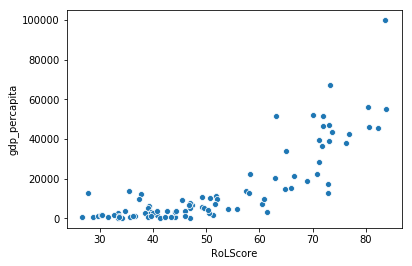What's going on here? If you look at this plot, it looks like rule of law scores are spread out across their range, while GDP seems to be clustered toward the bottom. Maybe we should inspect the distributions of the two variables?

In :
sns.distplot(df["RoLScore"])

Out:
<matplotlib.axes._subplots.AxesSubplot at 0x112220b70>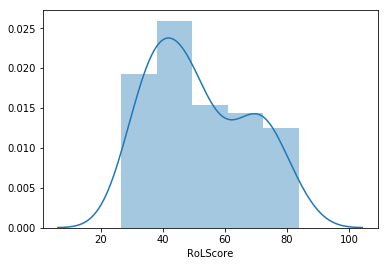In :
sns.distplot(df["gdp_percapita"])

Out:
<matplotlib.axes._subplots.AxesSubplot at 0x112358438>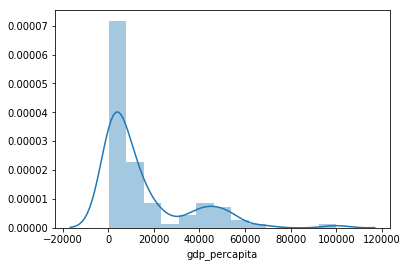So they're both obviously a bit skewed, but the rule of law is much less skewed. In fact, what we see in the GDP data is pretty common for most data involving money: there are way, way, way more points at the low end than at the higher end. Lots of poor countries, very few super-rich countries like the U.S. This often calls for a logarithmic transformation to understand the relationship between the two data points.

## Log Transformations¶

As you may or may not recall from high school math, taking a logarithm is the inverse of exponentiation. That is, the statement $2^3 = 8$ is equivalent to the statement $\log_2 8 = 3$.

Logarithms are a bit tough for human psychology to really have intuitions about, but one good way to think of them is that they are a way of turning differences across orders of magnitude to differences within a single magnitude.

That is, suppose we had the following data: [1, 10, 100, 1000, 10000, 100000, 1000000, 10000000]. The range goes from one to ten million and the difference between the first and the last element is obviously huge. But if we take the log base 10 of every element in there, we end up with much smaller differences and a much less curvy line representing our datapoints in a graph.

In :
from math import log10
toydata = [1, 10, 100, 1000, 10000, 100000, 1000000, 10000000]
loggedtoydata = [log10(x) for x in toydata]
print(loggedtoydata)

[0.0, 1.0, 2.0, 3.0, 4.0, 5.0, 6.0, 7.0]

In :
sns.scatterplot(range(0, 8), toydata)

Out:
<matplotlib.axes._subplots.AxesSubplot at 0x112430198>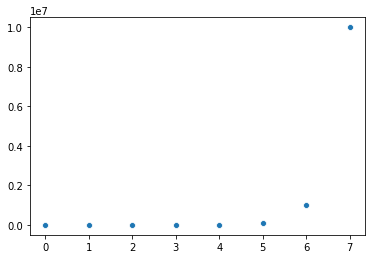In :
sns.scatterplot(range(0, 8), loggedtoydata)

Out:
<matplotlib.axes._subplots.AxesSubplot at 0x11250fc18>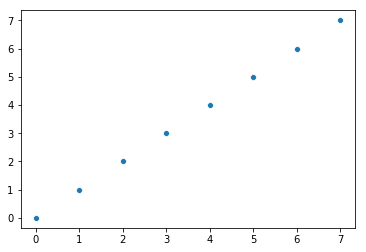So let's do that to our gdp to see if it helps us make an interpretable graph. (We'll have to use numpy log functions because they can apply to a series of data just as well as an individual point, so we'll just shadow (overwrite) the log10 name we originally imported from the Python standard library with one from numpy. This is normally a bad idea.)

In :
from numpy import log10
df["log_gdp"] = log10(df["gdp_percapita"])

In :
sns.scatterplot(df["RoLScore"], df["log_gdp"])

Out:
<matplotlib.axes._subplots.AxesSubplot at 0x11257cb70>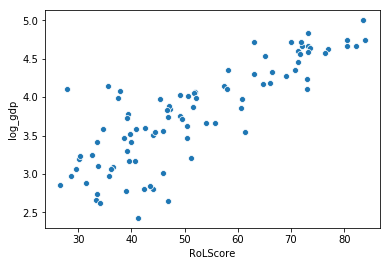What we can see is that there's actually a pretty clear relationship between rule of law scores and GDP per capita, after a log transformation. The way to read this graph is that as GDP per capita increases by a factor of 10, rule of law scores seem to increase by, oh, 15 points or thereabouts. This relationship is much harder to see on the original scatterplot.

Log-transforming data relating to money is often a good idea.

## Centering and Scaling¶

Centering and scaling is carried out as follows:

1. Subtract the mean of the variable from each of its values ("centering" --- this effectively converts the mean of the variable to zero)

2. Divide each variable by its standard deviation.

We can easily use more numpy functions to achieve this.

In :
from numpy import mean, std

def center_scale(variable):  # this applies to an entire pandas column
mu = mean(variable)
sigma = std(variable)
return (variable - mu) / sigma


What this will ultimately do is reduce a variable to what we might call standard deviation units. Let's look at another example. In the dataset we're working with, the variable hprop is the heritage foundation property rights score for 2012, and assoc_org is the Freedom House associational and organizational rights score. Observe:

In :
df[['State', 'hprop', 'assoc_org', 'RoLScore']].head(10)

Out:
State hprop assoc_org RoLScore
0 Albania 30 8 42.60
1 Argentina 15 11 51.94
2 Australia 90 12 73.28
3 Austria 90 12 73.15
5 Belgium 80 12 73.57
6 Bolivia 10 9 33.51
7 Bosnia and Herzegovina 20 7 55.77
8 Botswana 70 10 60.56
9 Brazil 50 10 51.83

As you can see, again, they're on different scales, which might make it a bit difficult to compare them, especially later on when we try to figure out the magnitude of their relationship with something else.

For example, suppose we learn from statistical analysis that a one-unit change in the Freedom House score predicts a one-unit change in the Rule of Law Score, but it takes a five-unit change in the Heritage Foundation score to predict a one-unit change in the Rule of Law Score. What does that mean in terms of the relative predictive power of those two variables? It's hard to tell, because they're not on a common scale. A one-unit change in the Freedom House score could be a really big difference, while a five-unit change in the Heritage Foundation score could be a really small difference. They're hard to compare.

Let's standardize, a.k.a. center and scale, them.

In :
df["hprop_std"] = center_scale(df["hprop"])
df["assoc_std"] = center_scale(df["assoc_org"])

Out:
State hprop_std assoc_std
0 Albania -0.694753 -0.208417
1 Argentina -1.289323 0.772582
2 Australia 1.683525 1.099582
3 Austria 1.683525 1.099582